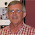Thursday, September 15, 2011

Micronumerosity

In a much earlier post I took a jab at the excessive attention paid to the concept of "multicollinearity", historically, in econometrics text books.

Art Goldberger (1930-2009) made numerous important contributions to econometrics, and modelling in the social sciences in general. He wrote several great texts, the earliest of which (Goldberger, 1964) was one of the very first to use the matrix notation that we now take as standard for the linear regression model.

In one of  his text books, Art also poked fun at the attention given to multicollinearity, and I'm going to share his parody with you here in full. In a couple of places I've had to replace formulae with words. What follows is from Chapter 23.3. of Goldberger (1991):

"Econometrics texts devote many pages to the problem of multicollinearity in multiple regression, but they say little about the closely analogous problem of small sample size in estimation a univariate mean. Perhaps that imbalance is attributable to the lack of an exotic polysyllabic name for 'small sample size'. If so, we can remove that impediment by introducing the term micronumerosity.

Suppose an econometrician set out to write a chapter about small sample size in sampling from a univariate population. Judging from what is now written about multicollinearity, the chapter might look like this:

1. Micronumerosity
The extreme case, 'exact micronumerosity', arises when n = 0; in which case the sample estimate of μ is not unique. (Technically, there is a violation of the rank condition n > 0: the matrix 0 is singular.) The extreme case is easy enough to recognize. 'Near micronumerosity' is more subtle, and yet very serious. It arises when the rank condition n > 0 is barely satisfied. Near micronumerosity is very prevalent in empirical economics.

2. Consequences of micronumerosity
The consequences of micronumerosity are serious. Precision of estimation is reduced. There are two aspects of this reduction: estimates of μ may have large errors, and not only that, but [the variance of the sample mean; DG] will be large.

Investigators will sometimes be led to accept the hypothesis μ = 0 because [the ratio of the sample mean to its standard error; DG] is small, even though the true situation may be not that μ = 0 but simply that the sample data have not enabled us to pick μ up.

The estimate of μ will be very sensitive to sample data, and the addition of a few more observations can sometimes produce drastic shifts in the sample mean.

The true μ may be sufficiently large for the null hypothesis μ= 0 to be rejected, even though [the variance of the sample mean; DG]  = σ2/n is large because of micronumerosity. But if the true μ is small (although nonzero) the hypothesis μ = 0 may mistakenly be accepted.

3. Testing for micronumerosity
Tests for the presence of micronumerosity require the judicious use of various fingers. Some researchers prefer a single finger, others use their toes, still others let their thumbs rule.

A generally reliable guide may be obtained by counting the number of observations. Most of the time in econometric analysis, when n is close to zero, it is also far from infinity.

Several test procedures develop critical values n*; such that micronumerosity is a problem only if n is smaller than n*: But those procedures are questionable.

4. Remedies for micronumerosity
If micronumerosity proves serious in the sense that the estimate of μ has an unsatisfactorily low degree of precision, we are in the statistical position of not being able to make bricks without straw. The remedy lies essentially in the acquisition, if possible, of larger samples from the same population.

But more data are no remedy for micronumerosity if the additional data are simply 'more of the same'. So obtaining lots of small samples from the same population will not help."

If you check the data that go with that earlier post of mine, you'll see that in this text book, Goldberger devoted 8 pages (2.12% of the book) - including what you've just read - to the topic of multicollinearity. The average for the introductory texts in my sample was 2.15%. This was probably the only time that Art was (slightly) below average!

References

Goldberger, A. S.  (1964). Econometric Theory. Wiley, New York.

Goldberger, A. S. (1991). A Course in Econometrics. Harvard University Press, Cambridge MA.

© 2011, David E. Giles

1.I think the reason multicollinearity is emphasised so much is because its easy to understand the problem - so people talk about it lot. (Makes people look knowledgeable when really they're not).

2.Really enjoyed this post, thank you. There can sometimes be a bit of a disconnect between the contents of stats/econometrics courses and the nitty gritty of empirical research...

3.Sinclair, Frances: Thanks for the comments!

4.in multiple regession, if two or more explanatory variables are highly correlated with each other, multicollinearity will occur. Is this the standard assumption ?

1.Assumption????? No!

2.Why is this not the case ?

3.Sorry - you've lost me! Why would we assume that the variables are collinear??

4.The question that was aked to me was: is the following statement correct "in multiple regession, if two or more explanatory variables are highly correlated with each other, multicollinearity will occur" explain your reasoning, I assumed that this statement would be correct? But now that you have said no I'm confused and I don't have great sources of information to guide me
Thanks for your time!

5.I didn't say "no"; I said why would you ASSUME that they are collinear. Of course, if the variable are highly correlated, multicollinearity will occur. That's just the definition of multicollinearity! What more can I say?

5.Thanks for sharing